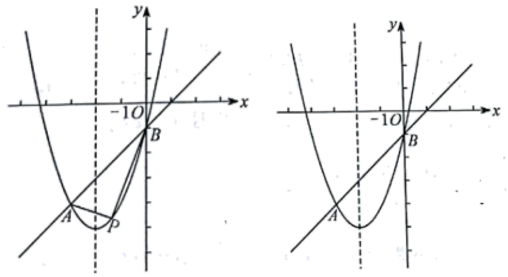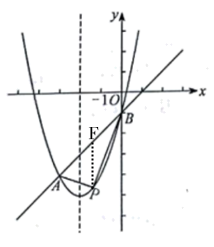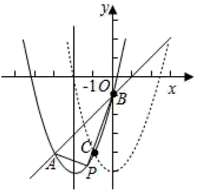（1）求该抛物线的函数表达式;
(2) 点 $\mathrm{P}$ 为直线 $\mathrm{AB}$ 下方抛物线上的任意一点, 连接 $\mathrm{PA}, \mathrm{PB}$, 求 $\triangle P A B$ 面积的最大值;
(3) 将该抛物线向右平移 2 个单位长度得到抛物线 $y=a_1 x^2+b_1 x+c_1\left(a_1 \neq 0\right)$, 平移后的抛物线与原抛物 线相交于点 $C$, 点 $D$ 为原抛物线对称轴上的一点, 在平面直角坐标系中是否存在点 $E$, 使以点 $B, C, D, E$ 为顶点的四边形为菱形, 若存在, 请直接写出点 $E$ 的坐标; 若不存在, 请说明理由.\begin{aligned} & \therefore\left\{\begin{array}{c} 9-3 b+c=-4 \\ c=-1 \end{array}\right. \\ & \therefore\left\{\begin{array}{c} b=4 \\ c=-1 \end{array}\right. \\ & \therefore y=x^2+4 x-1 \end{aligned}
(2) 设 $y_{A B}=k x+b$, 将点 $A(-3,-4) B(0,-1)$ 代入 $y_{A B}$
$$\therefore y_{A B}=x-1$$$$\begin{gathered} S_{\triangle P A B}=\frac{1}{2}|P F| \cdot\left|x_B-x_A\right| \\ =\frac{3}{2}\left(a-1-a^2-4 a+1\right) \\ =\frac{3}{2}\left(-a^2-3 a\right) \\ =-\frac{3}{2}\left(a+\frac{3}{2}\right)^2+\frac{27}{8} \end{gathered}$$

$\therefore \triangle P A B$ 面积最大值为 $\frac{27}{8}$
（3）(3）抛物线的表达式为: $y=x^2+4 x-1=(x+2) 2-5$, 则平移后的抛物线表达式为: $\mathrm{y}=\mathrm{x}^2-5$,(1) 当 $\mathrm{BC}$ 为菱形的边时,

(D),

(2)当 $\mathrm{BC}$ 为菱形的的对角线时,

$\therefore$ 存在, $E_1(-1,2), E_2(-3,-4+\sqrt{6}), E_3(-3,-4-\sqrt{6}), E_4(1,-3)$
【点睛】本题考查的是二次函数综合运用, 涉及到一次函数的性质、菱形的性质、图形的平移、面积的计 算等, 其中 (3), 要注意分类求解, 避免遗漏.
①点击 首页查看更多试卷和试题 , 点击查看 本题所在试卷